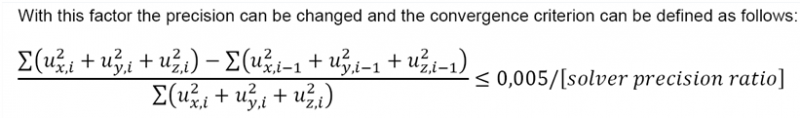# How to modify the solver precision?

There are some default tolerance values in many nonlinear analysis processes in the solver.

The "solver precision ratio" in solver settings (Setup -> Solver > Advanced solver settings > Nonlinearity) allows modifying these default tolerances.

The tolerances in the solver will be divided by the specified precision ratio.

For instance, a precision ratio of 10 means that all tolerance values will be 10 times smaller than the default setting (=higher precision).

Below you can find an example of a default tolerance (=convergence criterion) and how it can be influenced via the solver precision ratio.